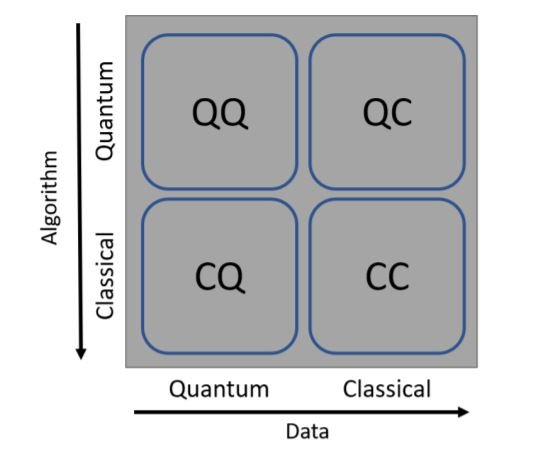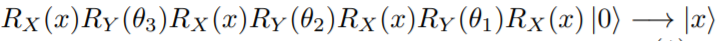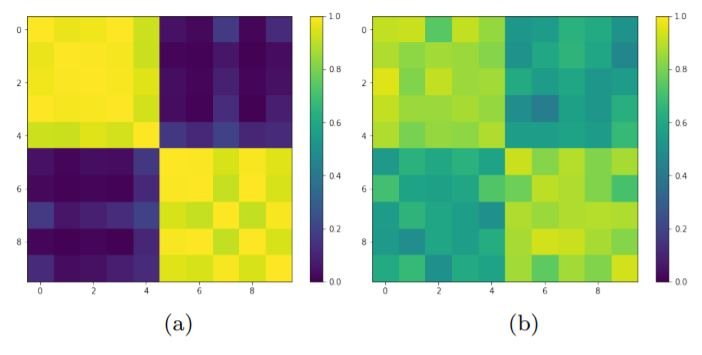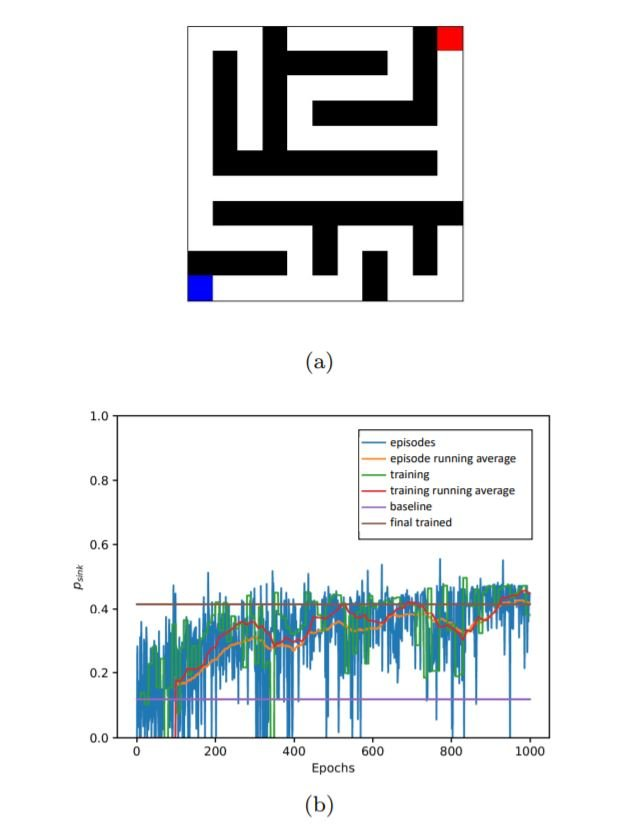# Catch up on the latest AI articles# What's Going On In Quantum Machine Learning?

3 main points
✔️ Quantum machine learning Recent developments in
✔️ Classical machine learning and quantum machine learning Comparison between
✔️ Emerging trends and future of quantum machine learning

written by Lorenzo BuffoniFilippo Caruso
(Submitted on 22 Aug 2021)

Subjects:  Quantum Physics (quant-ph); Disordered Systems and Neural Networks (cond-mat.dis-nn); Machine Learning (cs.LG); Machine Learning (stat.ML)code：ssue EPL Volume 132, Number 6, December 2020 Article Number 60004 Number of page(s) 7 Section General DOI https://doi.org/10.1209/0295-5075/132/60004

The images used in this article are from the paper, the introductory slides, or were created based on them.

## Introduction

Due to the potential that machine learning holds, it has today become a multidisciplinary field. ML systems are getting ever more powerful, and the difficulty of training and developing these systems is also increasing rapidly. This has increased researchers' interest in using quantum computing to perform machine learning (QML). Large and small tech companies have started investing in the development of quantum computers to perform ML on them.

However, quantum computing is challenging. Fault-tolerant quantum computers which require the integration of millions of qubits are hard to develop. Optimistically, there are several prospects to get powerful QML algorithms on currently available Noisy Intermediate Scale Quantum(NISQ) devices. In fact, several breakthroughs have already been made. In this paper, we explain new possible trends of QML in three main domains of machine learning: supervised learning, unsupervised learning, and reinforcement learning.

## Types of QMLML can be classified based on whether the data is classical or quantum, and on whether the ML algorithm used is classical or quantum. As shown in the above figure if either the data or algorithm or both are quantum in nature, the computation can be considered quantum computing: QQ, QC, CQ. This is not a strict classification, and there are also several hybrid algorithms in use. For example, in some cases, only the optimization task is carried out by a quantum processor and the rest by a classical one. However, we will stick to this classification throughout the paper.

### QML in Supervised Learning

There are several implementations of supervised learning algorithms for current NISQ devices. One of such approaches is to embed classical data into a larger quantum (Hilbert) space and make the classes easier to separate by a hyperplane. This approach is similar to classical support vector machines. The embedding is performed using a quantum circuit made of single and multi-qubit gates. Symbolically, mapping classical data x into a single qubit |x> can be represented by:where RX and RY are rotation operators along X and Y axes, and the rotation angles {θ1, θ2, θ3} are the trainable parameters of the model. Once all the data points are embedded,  the SWAP test is used to calculate the overlap between any two points. Points (states) of the same class have an overlap close to 1 while points from different classes have an overlap close to 0. Thus the dataset can be classified.As an example, the above diagram shows the theoretical Gram matrix(a) and experimental Gram matrix(b) for 10 validation points carried out on the IBM Valencia QPU (composed of 5 qubits). Despite some noise, we were able to achieve a good classification boundary between the classes. Currently, this method is effective on smaller datasets embedded in 1 or 2 qubits. Theoretically, a circuit of 100 qubits with circuit depth 100 and a decoherence time of 10−3s, could embed O(1010) bits of classical information, i.e. a task which is classically unattainable.

Once classical data is embedded in the quantum space, it becomes a challenge to discriminate among a set of non-orthogonal quantum states. A solution is to optimize a classical neural network to obtain the highest probability of correct discrimination between any two quantum states. But this probabilistic approach quickly approaches the theoretical limit called the Helstrom bound.

As a CQ-type application(quantum data and classical algorithm), artificial neural networks can be used for Quantum Noise Discrimination in stochastic quantum dynamics. ML algorithms like SVM, GRU, LSTMs are promising candidates in this field.

### QML in unsupervised learning

Unsupervised learning is much harder and therefore largely unresolved.

For clustering algorithms, a distance measure(like euclidean distance) is used to calculate the distance between any two points and create a distance matrix.  This matrix can also be interpreted as the adjacency matrix of a weighted graph G, and the problem of clustering reduces to a MAXCUT optimization problem on graph G. THe MAXCUT problem is NP-complete and very hard to solve. Hybrid approaches have been used to solve clustering problems by applying a quantum-based optimization scheme to a classical algorithm. The QAOA algorithm was able to solve the MAXCUT problem applied to a synthetic dataset composed of 2 clusters and 10 points in 2-dimensional space.

Variational Autoencoders(VAEs) are popular generative models that are able to learn a complex data distribution.  VAEs learn a probability distribution where usually the posterior distribution is implemented by a Deep Neural Network and the prior distribution is a simple distribution (e.g., i.i.d Gaussian or Bernoulli variables). It is a good idea to replace the simple prior with a more complex distribution sampled from a quantum device because offloading the generative capacity to the prior distribution by exploiting large graphs capable of representing complex probability distributions is classically too expensive.

GANs are another class of generative learning models. At a certain point, the discriminator and generator models reach a Nash equilibrium where the generator is able to exactly reproduce the desired (real) data distribution. Quantum GANs are an extension of classical GANs and an example of QQ-type QML. For QGANs, the goal is to learn to reproduce the state of a quantum physical system, e.g. a register of qubits.

### QML in reinforcement learning

RL can be generalized to QML to solve a QQ-type problem called the quantum maze problem. A quantum maze is a network whose topology is represented by a perfect maze i.e. there is a unique path between two edge points on the maze and the evolution of the state of the maze is quantum in nature. The paths of the maze are defined by links between nodes, which are described by an adjacency matrix A(Ai,j = 1 for presence of link, and 0 indicates absence). The goal is to maximize this escaping probability in the shortest amount of time.

The maze is the RL environment, and an external controller acts as the agent. The agent has some information about the quantum state of the system. The agent may also be allowed to change the state of the maze by building (Ai,j = 1), or destroying (Ai,j = 0) the walls of the maze. The state of the maze could also change intrinsically by random flips.The above diagrams show an example where an agent was trained to perform the described actions on a 6 × 6 perfect maze. The performance improves from the baseline stochastic quantum walker as the agent learns how to get better rewards by transferring more population to the end. This RL approach could help optimize the transport of energy and information over complex networks and lead to the development of better QML, NISQ technologies.

Summary

This paper provides valuable insights on recent trends in purely quantum(QQ) and classical-quantum hybrid algorithms and data. Quantum computing/quantum machine learning are still in their early stages of development. As pointed in the paper, the theoretical prospects of QML are numerous. However, current QML systems are resource-intensive and show subpar performance compared to classical ML systems. Much effort is necessary for the development of QML systems that are deployable in real-life scenarios.

If you have any suggestions for improvement of the content of the article,